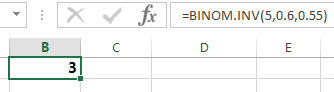## Excel BINOM.INV function

This post will guide you how to use the BINOM.INV function with syntax and examples in Microsoft excel.

### Description

The Excel BINOM.INV function returns the inverse of the Cumulative Binomial Distribution that is greater than or equal to a criterion value. And this function will return the smallest value for which the cumulative binomial distribution.

The BINOM.INV function is a build-in function in Microsoft Excel and it is categorized as a Statistical Function.

The BINOM.INV function is available in Excel 2016, Excel 2013, Excel 2010, Excel 2011 for Mac.

### Syntax

The syntax of the BINOM.INV function is as below:

`=BINOM.INV(trials, probability_s, alpha)`

Where the BINOM.INV function arguments are:

• Trials – This is a required argument.  The number of Bernoulli trials.
• probability_s – This is a required argument.  The probability of success for each trial.
• Alpha – This is a required argument. The criterion value. The value must be 0 or 1.

Note:

• If any argument is non-numeric, the BINOM.INV function will return #VALUE! Error.
• If trials argument is not integers and the BINOM.INV function will truncate it.
• If trials argument is smaller than 0, the BINOM.INV function will return the #NUM! Error.
• If probability_s is small than 0 or greater than 1, then the BINOM.INV function will return the #NUM! Error.
• If alpha argument is smaller than 0 or greater than 1, then the BINOM.INV function will return the #NUM! Error.

### Excel BINOM.INV Function Examples

The below examples will show you how to use Excel BINOM.INV Function to get the inverse of the Cumulative Binomial Distribution.

Example 1: to get the smallest value for which the cumulative binomial distribution is greater than or equal to a criterion value, using the following formula:

`= BINOM.INV (5,0.6,0.55)`### Related Functions

• Excel BINOMDIST function
The Excel BINOMDIST function returns the individual term binomial distribution probability. The syntax of the BINOMDIST function is as below:= BINOMDIST (number_s, trials, probability_s, cumulative).…
• Excel BINOM.DIST function
The Excel BINOM.DIST function returns the individual term binomial distribution probability. The syntax of the BINOM.DIST function is as below:= BINOM.DIST(number_s, trials, probability_s, cumulative).…